Publicité

# GRADE 8-ILLUSTRATING THE SAS, ASA AND SSS.pptx

23 Mar 2023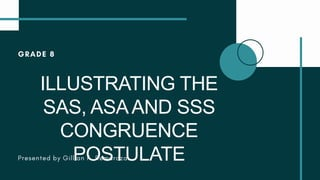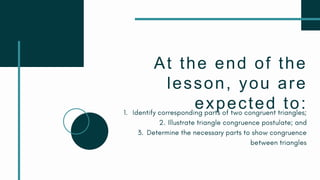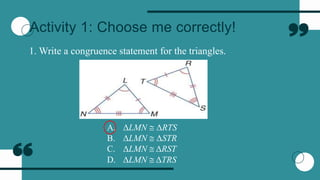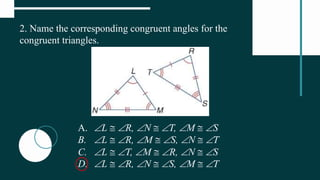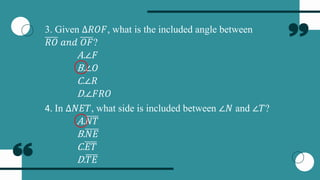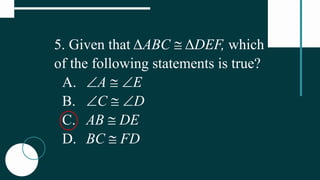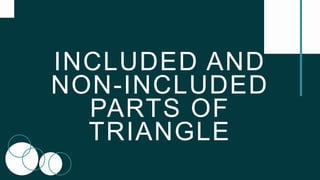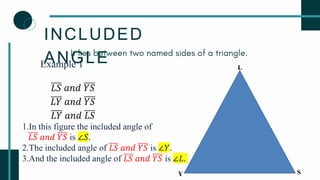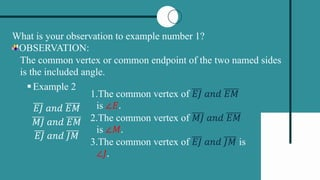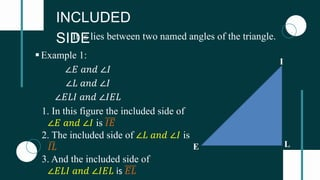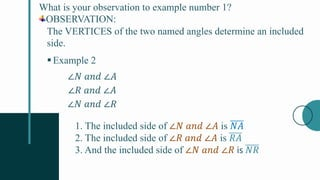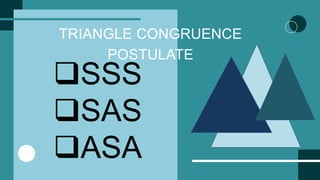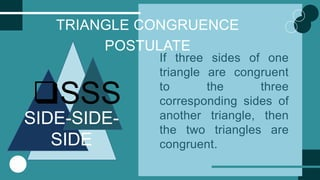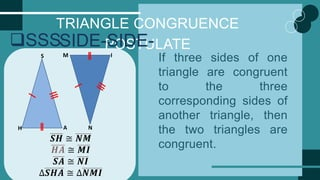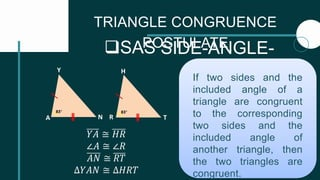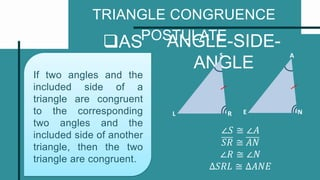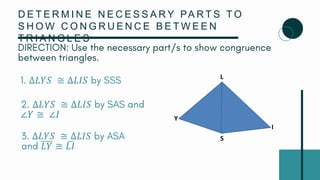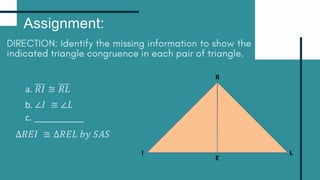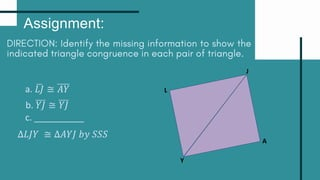1 sur 20
Publicité

### GRADE 8-ILLUSTRATING THE SAS, ASA AND SSS.pptx

1. ILLUSTRATING THE SAS, ASAAND SSS CONGRUENCE POSTULATE
2. At the end of the lesson, you are expected to:
3. Activity 1: Choose me correctly! 1. Write a congruence statement for the triangles. A. ΔLMN  ΔRTS B. ΔLMN  ΔSTR C. ΔLMN  ΔRST D. ΔLMN  ΔTRS
4. 2. Name the corresponding congruent angles for the congruent triangles. A. L  R, N  T, M  S B. L  R, M  S, N  T C. L  T, M  R, N  S D. L  R, N  S, M  T
5. 3. Given ∆𝑅𝑂𝐹, what is the included angle between 𝑅𝑂 𝑎𝑛𝑑 𝑂𝐹? A.∠𝐹 B.∠𝑂 C.∠𝑅 D.∠𝐹𝑅𝑂 4. In ∆𝑁𝐸𝑇, what side is included between ∠𝑁 and ∠𝑇? A.𝑁𝑇 B.𝑁𝐸 C.𝐸𝑇 D.𝑇𝐸
6. 5. Given that ΔABC  ΔDEF, which of the following statements is true? A. A  E B. C  D C. AB  DE D. BC  FD
7. INCLUDED AND NON-INCLUDED PARTS OF TRIANGLE
8. INCLUDED ANGLE L S Y Example 1 𝐿𝑆 𝑎𝑛𝑑 𝑌𝑆 𝐿𝑌 𝑎𝑛𝑑 𝑌𝑆 𝐿𝑌 𝑎𝑛𝑑 𝐿𝑆 1.In this figure the included angle of 𝐿𝑆 𝑎𝑛𝑑 𝑌𝑆 is ∠𝑆. 2.The included angle of 𝐿𝑆 𝑎𝑛𝑑 𝑌𝑆 is ∠𝑌. 3.And the included angle of 𝐿𝑆 𝑎𝑛𝑑 𝑌𝑆 is ∠𝐿.
9. What is your observation to example number 1? OBSERVATION: The common vertex or common endpoint of the two named sides is the included angle. Example 2 𝐸𝐽 𝑎𝑛𝑑 𝐸𝑀 𝑀𝐽 𝑎𝑛𝑑 𝐸𝑀 𝐸𝐽 𝑎𝑛𝑑 𝐽𝑀 1.The common vertex of 𝐸𝐽 𝑎𝑛𝑑 𝐸𝑀 is ∠𝐸. 2.The common vertex of 𝑀𝐽 𝑎𝑛𝑑 𝐸𝑀 is ∠𝑀. 3.The common vertex of 𝐸𝐽 𝑎𝑛𝑑 𝐽𝑀 is ∠𝐽.
10. INCLUDED SIDE It is lies between two named angles of the triangle. L E I ∠𝐸 𝑎𝑛𝑑 ∠𝐼 ∠𝐿 𝑎𝑛𝑑 ∠𝐼 ∠𝐸𝐿𝐼 𝑎𝑛𝑑 ∠𝐼𝐸𝐿 Example 1: 1. In this figure the included side of ∠𝐸 𝑎𝑛𝑑 ∠𝐼 is 𝐼𝐸 2. The included side of ∠𝐿 𝑎𝑛𝑑 ∠𝐼 is 𝐼𝐿 3. And the included side of ∠𝐸𝐿𝐼 𝑎𝑛𝑑 ∠𝐼𝐸𝐿 is 𝐸𝐿
11. What is your observation to example number 1? OBSERVATION: The VERTICES of the two named angles determine an included side. Example 2 ∠𝑁 𝑎𝑛𝑑 ∠𝐴 ∠𝑅 𝑎𝑛𝑑 ∠𝐴 ∠𝑁 𝑎𝑛𝑑 ∠𝑅 1. The included side of ∠𝑁 𝑎𝑛𝑑 ∠𝐴 is 𝑁𝐴 2. The included side of ∠𝑅 𝑎𝑛𝑑 ∠𝐴 is 𝑅𝐴 3. And the included side of ∠𝑁 𝑎𝑛𝑑 ∠𝑅 is 𝑁𝑅
12. TRIANGLE CONGRUENCE POSTULATE SSS SAS ASA
13. TRIANGLE CONGRUENCE POSTULATE SSS SIDE-SIDE- SIDE If three sides of one triangle are congruent to the three corresponding sides of another triangle, then the two triangles are congruent.
14. TRIANGLE CONGRUENCE POSTULATE SSSSIDE-SIDE- SIDE If three sides of one triangle are congruent to the three corresponding sides of another triangle, then the two triangles are congruent. 𝑺𝑯 ≅ 𝑵𝑴 𝑯𝑨 ≅ 𝑴𝑰 𝑺𝑨 ≅ 𝑵𝑰 ∆𝑺𝑯𝑨 ≅ ∆𝑵𝑴𝑰 S H A N M I
15. TRIANGLE CONGRUENCE POSTULATE SAS SIDE-ANGLE- SIDE If two sides and the included angle of a triangle are congruent to the corresponding two sides and the included angle of another triangle, then the two triangles are congruent. A Y N R H T 83° 83° 𝑌𝐴 ≅ 𝐻𝑅 ∠𝐴 ≅ ∠𝑅 𝐴𝑁 ≅ 𝑅𝑇 ∆𝑌𝐴𝑁 ≅ ∆𝐻𝑅𝑇
16. TRIANGLE CONGRUENCE POSTULATE AS A ANGLE-SIDE- ANGLE If two angles and the included side of a triangle are congruent to the corresponding two angles and the included side of another triangle, then the two triangle are congruent. ∠𝑆 ≅ ∠𝐴 𝑆𝑅 ≅ 𝐴𝑁 ∠𝑅 ≅ ∠𝑁 ∆𝑆𝑅𝐿 ≅ ∆𝐴𝑁𝐸 N E A L R S
17. D E T E R M I N E N E C E S S A R Y PA R T S T O S H O W C O N G R U E N C E B E T W E E N T R I A N G L E S ∆𝐿𝑌𝑆 ≅ ∆𝐿𝐼𝑆 ∆𝐿𝑌𝑆 ≅ ∆𝐿𝐼𝑆 ∠𝑌 ≅ ∠𝐼 ∆𝐿𝑌𝑆 ≅ ∆𝐿𝐼𝑆 𝐿𝑌 ≅ 𝐿𝐼 S I L Y
18. Assignment: R L I E a. 𝑅𝐼 ≅ 𝑅𝐿 b. ∠𝐼 ≅ ∠𝐿 c. __________ ∆𝑅𝐸𝐼 ≅ ∆𝑅𝐸𝐿 𝑏𝑦 𝑆𝐴𝑆
19. Assignment: a. 𝐿𝐽 ≅ 𝐴𝑌 c. __________ ∆𝐿𝐽𝑌 ≅ ∆𝐴𝑌𝐽 𝑏𝑦 𝑆𝑆𝑆 L A Y J b. 𝑌𝐽 ≅ 𝑌𝐽
20. THANK YOU!
Publicité